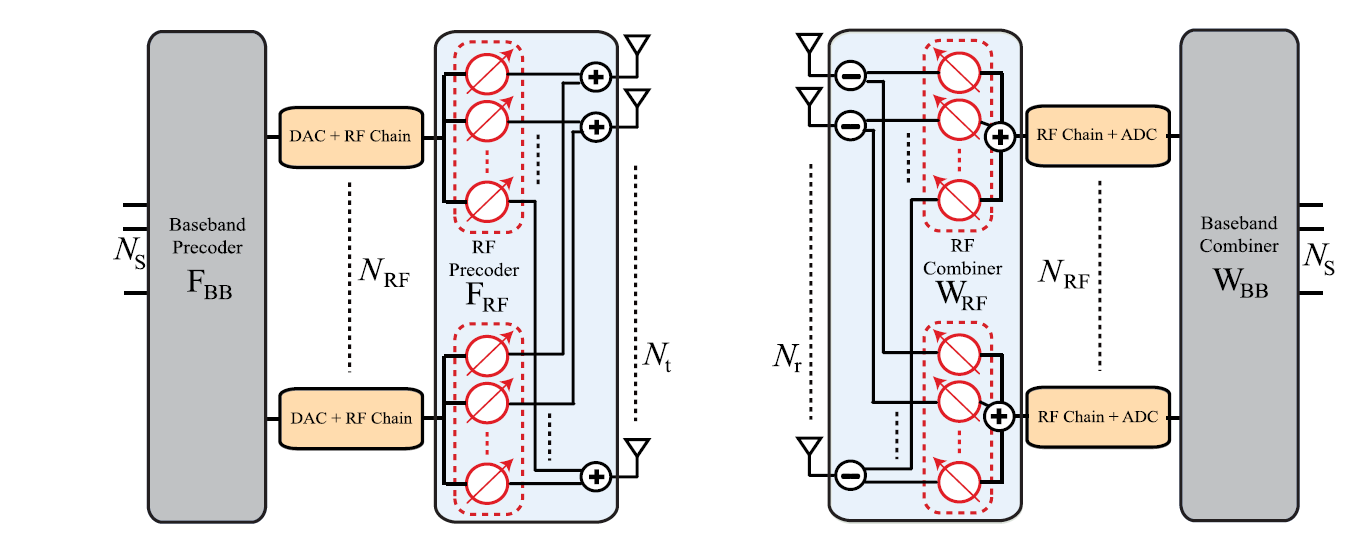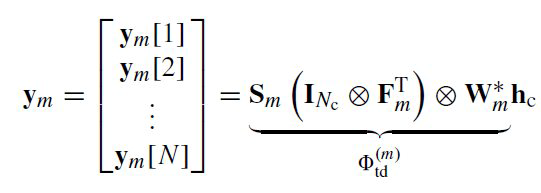# 简介

## 系统结构$\mathbf{y}[n]=\sqrt{\rho} \sum_{d=0}^{N_{c}-1} \mathbf{W}^{*} \mathbf{H}_{d} \mathbf{F} \mathbf{s}[n-d]+\mathbf{W}^{*} \mathbf{v}[n]$
$\mathbf{W}$$\mathbf{F}$分别是发送端和接收端的波束成形矩阵。

$\mathbf{H}_{d}=\sum_{\ell=1}^{N_{\mathrm{p}}} \alpha_{\ell} p\left(d T_{\mathrm{s}}-\tau_{\ell}\right) \mathbf{a}_{\mathrm{R}}\left(\phi_{\ell}\right) \mathbf{a}_{\mathrm{T}}^{*}\left(\theta_{\ell}\right)$

$\mathbf{H}_{d}=\mathbf{A}_{\mathrm{R}} \Delta_{d} \mathbf{A}_{\mathrm{T}}^{*}$

$\operatorname{vec}\left(\mathbf{H}_{d}\right)=\left(\overline{\mathbf{A}}_{\mathrm{T}} \circ \mathbf{A}_{\mathrm{R}}\right)\left[\begin{array}{c}{\alpha_{1} p\left(d T_{\mathrm{s}}-\tau_{1}\right)} \\ {\alpha_{2} p\left(d T_{\mathrm{s}}-\tau_{2}\right)} \\ {\vdots} \\ {\alpha_{N_{\mathrm{p}}} p\left(d T_{\mathrm{s}}-\tau_{N_{\mathrm{p}}}\right)}\end{array}\right]$

## 时域信道估计算法

$\mathbf{r}_{m}[n]=\sum_{d=0}^{N_{c}-1} \mathbf{H}_{d} \mathbf{F}_{m} \mathbf{s}_{m}[n-d]+\mathbf{v}_{m}[n]$

$\mathbf{s}_{m}=\left[\underbrace{0 \cdots 0}_{N_{\mathrm{c}}-1} \mathbf{s}_{m} \cdots \mathbf{s}_{m}[N]\right]$
$N_c$个为零前缀（ZP），用于防止相邻帧之间的干扰。

$\mathbf{y}_{m}=\left[\begin{array}{c}{\mathbf{y}_{m}} \\ {\mathbf{y}_{m}} \\ {\vdots} \\ {\mathbf{y}_{m}[N]}\end{array}\right]=\underbrace{\mathbf{S}_{m}\left(\mathbf{I}_{N_{c}} \otimes \mathbf{F}_{m}^{\mathrm{T}}\right) \otimes \mathbf{W}_{m}^{*} \mathbf{h}_{\mathbf{c}}+\mathbf{e}_{m}}$

$\mathbf{S}_{m}=\left[\begin{array}{cccc}{\mathbf{s}_{m}^{\mathrm{T}}} & {0} & {\cdots} & {0} \\ {\mathbf{s}_{m}^{\mathrm{T}}} & {\mathbf{s}_{m}^{\mathrm{T}}} & {\cdots} & {\cdot} \\ {\vdots} & {\vdots} & {\ddots} & {\vdots} \\ {\mathbf{s}_{m}^{\mathrm{T}}[N]} & {\cdots} & {\cdots} & {\mathbf{s}_{m}^{\mathrm{T}}\left[N-N_{\mathrm{c}}+1\right]}\end{array}\right]$

$\operatorname{vec}(\mathbf{A B C})=\left(\mathbf{C}^{\mathrm{T}} \otimes \mathbf{A}\right) \operatorname{vec}(\mathbf{B})$$\mathbf{y}_{m}=\Phi_{\mathrm{td}}^{(m)}\left(\mathbf{I}_{N_{\mathrm{c}}} \otimes \overline{\mathbf{A}}_{\mathrm{T}} \circ \mathbf{A}_{\mathrm{R}}\right)\left[\begin{array}{c}{\gamma_{1,0}} \\ {\vdots} \\ {\gamma_{N_{\mathrm{p}}, 0}} \\ {\vdots} \\ {\gamma_{1,\left(N_{\mathrm{c}}-1\right)}} \\ {\vdots} \\ {\gamma_{N_{\mathrm{p}}},\left(N_{\mathrm{c}}-1\right)}\end{array}\right]+\mathbf{e}$

$\mathbf{y}_{m}=\Phi_{\mathrm{td}}^{(m)}\left(\mathbf{I}_{N_{\mathrm{c}}} \otimes \overline{\mathbf{A}}_{\mathrm{tx}} \otimes \mathbf{A}_{\mathrm{rx}}\right) \hat{\mathbf{x}}_{\mathrm{td}}+\mathbf{e}_{m}$

$\mathbf{y}_{m}=\Phi_{\mathrm{td}}^{(m)}\left(\mathbf{I}_{N_{\mathrm{c}}} \otimes \overline{\mathbf{A}}_{\mathrm{tx}} \otimes \mathbf{A}_{\mathrm{rx}}\right) \Gamma \mathbf{x}_{\mathrm{td}}+\mathbf{e}_{m}$

$\Gamma=\left[\begin{array}{c}{\mathbf{I}_{G_{\mathrm{r}} G_{\mathrm{t}}} \otimes \mathbf{p}_{1}^{\mathrm{T}}} \\ {\mathbf{I}_{G_{\mathrm{r}} G_{\mathrm{t}}} \otimes \mathbf{p}_{2}^{\mathrm{T}}} \\ {\vdots} \\ {\mathbf{I}_{G_{\mathrm{r}} G_{\mathrm{t}}} \otimes \mathbf{p}_{N_{\mathrm{c}}}^{\mathrm{T}}}\end{array}\right]$

$\Phi_{\mathrm{td}}=\left[\begin{array}{c}{\mathbf{S}_{1}\left(\mathbf{I}_{N_{\mathrm{c}}} \otimes \mathbf{F}_{\mathrm{f}}^{\mathrm{T}}\right) \otimes \mathbf{W}_{1}^{*}} \\ {\mathbf{S}_{2}\left(\mathbf{I}_{N_{\mathrm{c}}} \otimes \mathbf{F}_{2}^{\dagger}\right) \otimes \mathbf{W}_{2}^{*}} \\ {\vdots} \\ {\mathbf{S}_{M}\left(\mathbf{I}_{N_{\mathrm{c}}} \otimes \mathbf{F}_{M}^{\mathrm{T}}\right) \otimes \mathbf{W}_{M}^{*}}\end{array}\right] \in \mathbb{C}^{N M N_{\mathrm{RF}} \times N_{\mathrm{c}} N_{\mathrm{r}} N_{\mathrm{t}}}$

$\Psi_{\mathrm{td}}=\left(\mathbf{I}_{N_{\mathrm{c}}} \otimes \overline{\mathbf{A}}_{\mathrm{tx}} \otimes \mathbf{A}_{\mathrm{rx}}\right) \Gamma$

$\mathbf{y}_{\mathrm{td}}=\Phi_{\mathrm{td}} \Psi_{\mathrm{td}} \mathbf{x}_{\mathrm{td}}+\mathbf{e}$

$\min _{\mathbf{x}_{\mathrm{td}}}\left\|\mathbf{x}_{\mathrm{td}}\right\|_{1} \quad \text { such that } \quad\left\|\mathbf{y}_{\mathrm{td}}-\Phi_{\mathrm{td}} \Psi_{\mathrm{td}} \mathbf{x}_{\mathrm{td}}\right\|_{2} \leq \epsilon$

OMP算法可以用于解决此类问题，如果信道的径数$l$已知，可以直接做$l$次迭代求解。 如果未知，则迭代到一定范围后停止。一般取，$\epsilon=\mathbb{E}\left[\mathbf{e}^{*} \mathbf{e}\right]$

## 频域估计方法

$\breve{y}_{m}[k]=\mathbf{W}_{m}^{*} \boldsymbol{H}[k] \mathbf{F}_{m} \breve{\boldsymbol{s}}_{m}[k]+\breve{\boldsymbol{e}}_{m}[k]$
$k$ 代表的是子载波index。

$H[k]=\sum_{\ell=1}^{N_{\mathrm{p}}} \alpha_{\ell} \beta_{k, \ell} \mathbf{a _ { \mathrm { R } }}\left(\phi_{\ell}\right) \mathbf{a}_{\mathrm{T}}^{*}\left(\theta_{\ell}\right)$

$\operatorname{vec}\left(\breve{\boldsymbol{y}}_{m}[k]\right)=\Phi_{\mathrm{fd}}^{(m)}[k]\left(\overline{\mathbf{A}}_{\mathrm{tx}} \otimes \mathbf{A}_{\mathrm{rx}}\right) \check{x}[k]+\breve{e}_{m}[k]$

## 结合估计方法

©️2019 CSDN 皮肤主题: 大白 设计师: CSDN官方博客